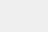# TensorFlow系列专题（六）：实战项目Mnist手写数据集识别

## 2． MNIST数据集

MNIST 数据集可以从网站 http://yann.lecun.com/exdb/mnist/ 上下载，需要下载的数据集总共有4个文件，其中”train-images-idx3-ubyte.gz”是训练集的图片，总共有60000张，”train-labels-idx1-ubyte.gz”是训练集图片对应的类标（0~9）。”t10k-images-idx3-ubyte.gz”是测试集的图片，总共有10000张，”t10k-labels-idx1-ubyte.gz”是测试集图片对应的类标（0~9）。TensorFlow的示例代码中已经对MNIST数据集的处理进行了封装，但是作为第一个程序，我们希望带着读者从数据处理开始做，数据处理在整个机器学习项目中是很关键的一个环节，因此有必要在第一个项目中就让读者体会到它的重要性。## 3． 数据处理

[python]
import numpy as np
import struct
import random

class MnistData:
def __init__(self, train_image_path, train_label_path,
test_image_path, test_label_path):
# 训练集和测试集的文件路径
self.train_image_path = train_image_path
self.train_label_path = train_label_path
self.test_image_path = test_image_path
self.test_label_path = test_label_path

# 获取训练集和测试集数据
# get_data()方法，参数为0获取训练集数据，参数为1获取测试集
self.train_images, self.train_labels = self.get_data(0)
self.test_images, self.test_labels = self.get_data(1)

# 定义两个辅助变量，用来判断一个回合的训练是否完成
self.num_of_batch = 0
self.got_batch = 0
[/python]

[python]
def get_data(self, data_type):
if data_type == 0: # 获取训练集数据
image_path = self.train_image_path
label_path = self.train_label_path
else: # 获取测试集数据
image_path = self.test_image_path
label_path = self.test_label_path

with open(image_path, ‘rb’) as file1:
with open(label_path, ‘rb’) as file2:

label_index = 0
image_index = 0
labels = []
images = []

# 读取训练集图像数据文件的文件信息
magic, num_of_datasets, rows, columns =\
struct.unpack_from(‘>IIII’, image_file, image_index)
image_index += struct.calcsize(‘>IIII’)

for i in range(num_of_datasets):
# 读取784个unsigned byte，即一副图像的所有像素值
temp = struct.unpack_from(‘>784B’, image_file, image_index)
# 将读取的像素数据转换成28*28的矩阵
temp = np.reshape(temp, (28, 28))
# 归一化处理
temp = temp / 255
images.append(temp)
image_index += struct.calcsize(‘>784B’) # 每次增加784B

# 跳过描述信息
label_index += struct.calcsize(‘>II’)
labels = struct.unpack_from(‘>’ + str(num_of_datasets)
+ ‘B’, label_file, label_index)

# one-hot
labels = np.eye(10)[np.array(labels)]

return images, labels
[/python]

[python]
def get_batch(self, batch_size):
# 刚开始训练或当一轮训练结束之后，打乱数据集数据的顺序
if self.got_batch == self.num_of_batch:
train_list = list(zip(self.train_images, self.train_labels))
random.shuffle(train_list)
self.train_images, self.train_labels = zip(*train_list)

# 重置两个辅助变量
self.num_of_batch = 60000 / batch_size
self.got_batch = 0

# 获取一个batch size的训练数据
train_images = self.train_images[
self.got_batch*batch_size:(self.got_batch+1)*batch_size]
train_labels = self.train_labels[
self.got_batch*batch_size:(self.got_batch+1)*batch_size]
self.got_batch += 1

return train_images, train_labels
[/python]

##4． 单层隐藏层神经网络的实现[python]
from mnist_data import MnistData
import tensorflow as tf
[/python]

[python]
# 创建Session会话
sess = tf.InteractiveSession()

# 训练集、测试集的文件路径
train_image_path = ‘./data/train-images-idx3-ubyte’
train_label_path = ‘./data/train-labels-idx1-ubyte’
test_image_path = ‘./data/t10k-images-idx3-ubyte’
test_label_path = ‘./data/t10k-labels-idx1-ubyte’

epochs = 10 # 训练的总轮数
batch_size = 100 # 每个batch的大小
learning_rate = 0.2 # 学习率
[/python]

“epochs”是我们想要训练的总轮数，每一轮都会使用训练集的所有数据去训练一遍模型。由于我们使用随机梯度下降方法更新参数，所以不会一次把所有的数据送进模型去训练，而是按批次训练，”batch_size”是我们定义的一个批次的数据量的大小，这里我们设定了100，那么每个”batch”就会送100个样本到模型中去训练，一轮训练的”batch”数等于总的训练集数量除以”batch_size”。”learning_rate”是我们定义的学习率，即模型参数更新的速率。

[python]
# 创建样本数据的placeholder
x = tf.placeholder(tf.float32, [None, 28, 28])
# 定义权重矩阵和偏置项
W = tf.Variable(tf.zeros([28*28, 10]))
b = tf.Variable(tf.zeros())

# 样本的真实标签
y_ = tf.placeholder(tf.float32, [None, 10])
# 使用softmax函数将单层网络的输出转换为预测结果
y = tf.nn.softmax(tf.matmul(tf.reshape(x, [-1, 28*28]), W) + b)
[/python]

[python]
# 损失函数和优化器
# -tf.reduce_sum(y_ * tf.log(y) 计算这个batch中每个样本的交叉熵
# reduce_mean方法对一个batch的样本的交叉熵求平均值，作为最终的loss
cross_entropy = tf.reduce_mean(-tf.reduce_sum(y_ * tf.log(y), axis=1))
train_step = \
[/python]

[python]
# 比较预测结果和真实类标
correct_prediction = tf.equal(tf.argmax(y, 1), tf.argmax(y_, 1))
# 计算准确率
accuracy = tf.reduce_mean(tf.cast(correct_prediction, tf.float32))
[/python]

“tf.equal()”方法用于比较两个矩阵或向量相应位置的元素是否相等，相等为”True”，不等为”False”。”tf.cast”用于将”True”和”False”转换为”1″和”0″，”tf.reduce_mean”对转换后的数据求平均值，该值即为模型在测试集上预测结果的准确率。最后，我们实现模型的训练和预测：

[python]
# 初始化MnistData类
data = MnistData(train_image_path, train_label_path,
test_image_path, test_label_path)
# 初始化模型参数
init = tf.global_variables_initializer().run()
# 开始训练
for i in range(epochs):
for j in range(600):
# 获取一个batch的数据
batch_x, batch_y = data.get_batch(batch_size)
# 优化参数
train_step.run({x: batch_x, y_: batch_y})
# 对测试集进行预测并计算准确率
print(accuracy.eval({x: data.test_images, y_: data.test_labels}))
[/python]

## 5． 多层神经网络的实现[python]
# 定义权重矩阵和偏置项
w_1 = tf.Variable(tf.truncated_normal([28*28, 200], stddev=0.1))
b_1 = tf.Variable(tf.zeros())
w_2 = tf.Variable(tf.truncated_normal([200, 10], stddev=0.1))
b_2 = tf.Variable(tf.zeros())
[/python]

[python]
# 定义一个两层神经网络模型
y_1 = tf.nn.sigmoid(tf.matmul(tf.reshape(x, [-1, 28*28]), w_1) + b_1)
y = tf.nn.softmax(tf.matmul(y_1, w_2) + b_2)
[/python]